# 14-1100/Homework Assignment 2

Jump to: navigation, search

Bad news. This assignment is due at class time on Thursday, October 16, 2010.

Good news. It will be marked by the following day, Friday October 17, and be available for pickup at my office between 2-4PM, providing you timely feedback for the term test on the following Monday.

### Solve the following problems

(but submit only your solutions of problems 2, 3, 4, 8, 9, and 11).

Problem 1. (Selick)

1. What it the least integer$n$ for which the symmetric group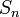$S_n$ contains an element of order 18?
2. What is the maximal order of an element in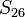$S_{26}$? (That is, of a shuffling of the red cards within a deck of cards?)

Problem 2. (Selick) Let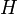$H$ be a subgroup of index 2 in a group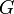$G$. Show that$H$ is normal in$G$.

Problem 3. Let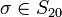$\sigma\in S_{20}$ be a permutation whose cycle decomposition consists of one 5-cycle, two 3-cycles, and one 2-cycle. What is the order of the centralizer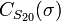$C_{S_{20}}(\sigma)$ of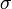$\sigma$?

Problem 4. (Selick) Let$G$ be a group of odd order. Show that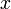$x$ is not conjugate to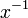$x^{-1}$ unless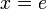$x=e$.

Problem 5. (Dummit and Foote) Show that if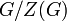$G/Z(G)$ is cyclic then$G$ is Abelian.

Problem 6. (Lang) Prove that if the group of automorphisms of a group$G$ is cyclic, then$G$ is Abelian.

Problem 7. (Lang)

1. Let$G$ be a group and let$H$ be a subgroup of finite index. Prove that there is a normal subgroup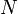$N$ of$G$, contained in$H$, so that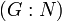$(G:N)$ is also finite. (Hint: Let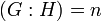$(G:H)=n$ and find a morphism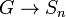$G\to S_n$ whose kernel is contained in$H$.)
2. Let$G$ be a group and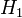$H_1$ and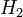$H_2$ be subgroups of$G$. Suppose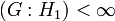$(G:H_1)<\infty$ and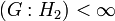$(G:H_2)<\infty$. Show that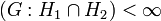$(G:H_1\cap H_2)<\infty$

Problem 8. (Selick) Show that any group of order 56 has a normal Sylow-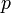$p$ subgroup, for some prime$p$ dividing 56.

Problem 9. (Qualifying exam, May 1997) Let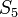$S_5$ act on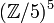$({\mathbb Z/5})^5$ by permuting the factors, and let$G$ be the semi-direct product of$S_5$ and$({\mathbb Z/5})^5$.

1. What is the order of$G$?
2. How many Sylow-5 subgroups does$G$ have? Write down one of them.

Problem 10. (Selick) Show that the group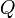$Q$ of unit quaternions (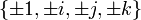$\{\pm 1, \pm i, \pm j, \pm k\}$, subject to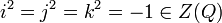$i^2=j^2=k^2=-1\in Z(Q)$ and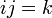$ij=k$) is not a semi-direct product of two of its proper subgroups.

Problem 11. (Qualifying exam, September 2008) Let$G$ be a finite group and$p$ be a prime. Show that if$H$ is a$p$-subgroup of$G$, then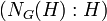$(N_G(H):H)$ is congruent to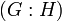$(G:H)$ mod$p$. You may wish to study the action of$H$ on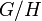$G/H$ by multiplication on the left.